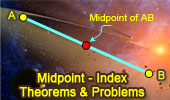# Midpoint, Theorems and Problems - Table of Content (Page 10)Generalizing Van Aubel: Michael de Villiers' Theorems.
Midpoints.

Proposed Problem 47. Angles and triangles.
Midpoints.

Proposed Problem 46. Angles and triangles. Midpoints.

Triangle and Squares 16
Midpoints.

Triangle and Squares 12.
Midpoints.

Triangle and Squares 11.
Midpoints.

Triangle and Squares 7
Midpoints.

Triangle and Squares 5
Midpoints.

Triangle and Squares 4
Midpoints.

Triangle and Squares 2
Midpoints.

Go to Page: Previous | 1 | 2 | 3 | 4 | 5 | 6 | 7 | 8 | 9 | 10 | Next

 Home | Search | Geometry | Post a comment | Email | by Antonio Gutierrez